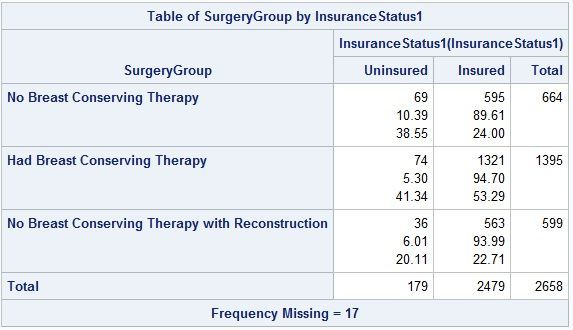## A calculated percentage from PROC FREQ or other method...

I have a frequency table given by PROC FREQ that looks like this:

```PROC FREQ DATA = have;
TABLES SurgeryGroup * InsuranceStatus / nopercent;
RUN;```

It gives me the correct table that looks like this:I would like to make a separate calculation off of this table called the "Reconstruction Rate" - subdivided by insurance status like above - which is basically:

No BCT w/ Recon / (No BCT w/ Recon + No BCT)

Example: 36/(36 + 69)     &      563/(563 + 595)

It should be coded so that if the frequencies of the table change (possibly due to more data added at a later date), then so does the answer to the calculation.

Any help is appreciated. Thank you!

1 ACCEPTED SOLUTION

Accepted Solutions

## Re: A calculated frequency from PROC FREQ or other method...

Create an output data set, and then do the additional calculations using this output data set from PROC FREQ.

To create an output data set, use

``TABLES SurgeryGroup * InsuranceStatus / nopercent out=results;``

OR

If I am udnerstanding the additional calculations correctly, you could run another PROC FREQ wihtout the category "Had Breast Conserving Surgery" and then this second PROC FREQ would give you the results directly.

--
Paige Miller

## Re: A calculated frequency from PROC FREQ or other method...

Create an output data set, and then do the additional calculations using this output data set from PROC FREQ.

To create an output data set, use

``TABLES SurgeryGroup * InsuranceStatus / nopercent out=results;``

OR

If I am udnerstanding the additional calculations correctly, you could run another PROC FREQ wihtout the category "Had Breast Conserving Surgery" and then this second PROC FREQ would give you the results directly.

--
Paige Miller
Discussion stats